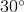Angle

While playing a game with friends, you are using a spinner. You know that the best number to land on is. The spinner looks like this: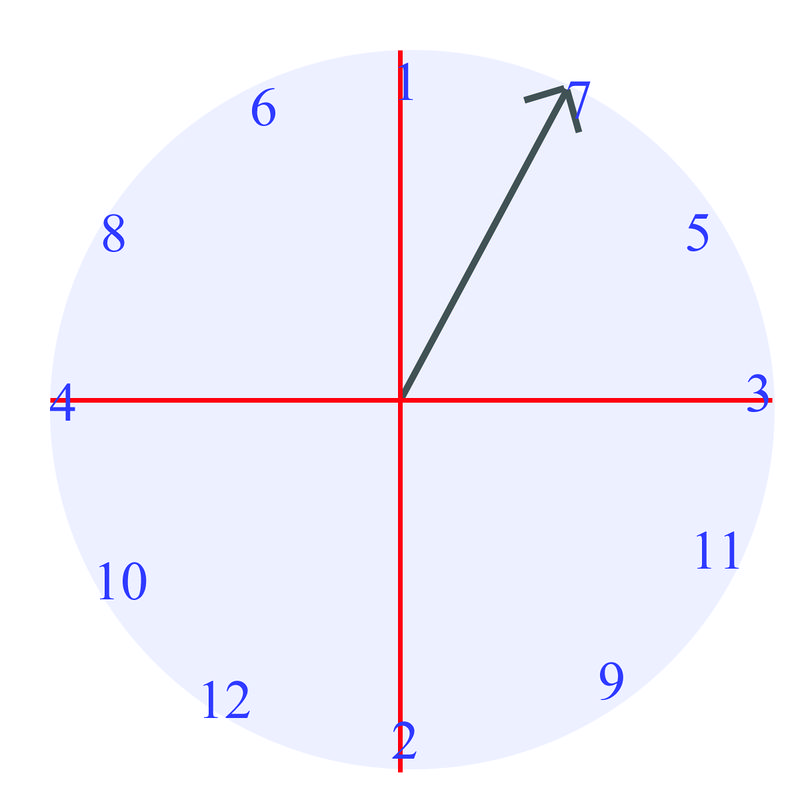Can you determine how to represent the angle of the spinner if it lands on the? Read on to answer this question by the end of this Concept.

Guidance

Consider our game that is played with a spinner. When you spin the spinner, how far has it gone? You can answer this question in several ways. You could say something like “the spinner spun around 3 times.” This means that the spinner made 3 complete rotations, and then landed back where it started.

We can also measure the rotation in degrees. In the previous lesson we worked with angles in triangles, measured in degrees. You may recall from geometry that a full rotation is 360 degrees, usually written as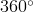. Half a rotation is thenand a quarter rotation is. Each of these measurements will be important in this Concept. We can use our knowledge of graphing to represent any angle. The figure below shows an angle in what is called standard position.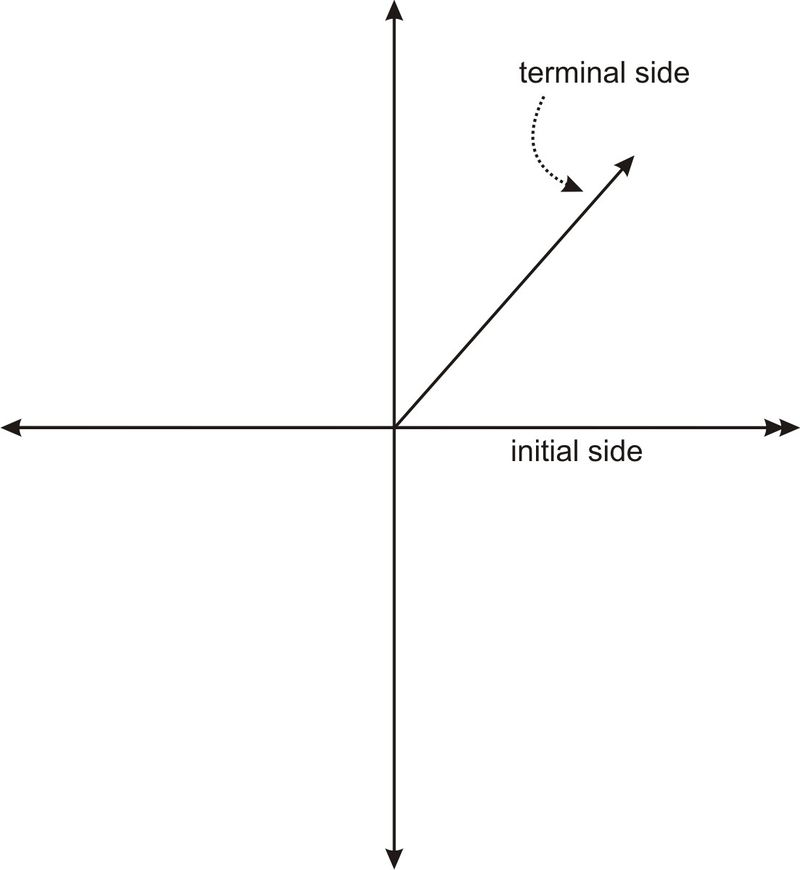The initial side of an angle in standard position is always on the positiveaxis. The terminal side always meets the initial side at the origin. Notice that the rotation goes in acounterclockwise direction. This means that if we rotate clockwise, we will generate a negative angle. Below are several examples of angles in standard position.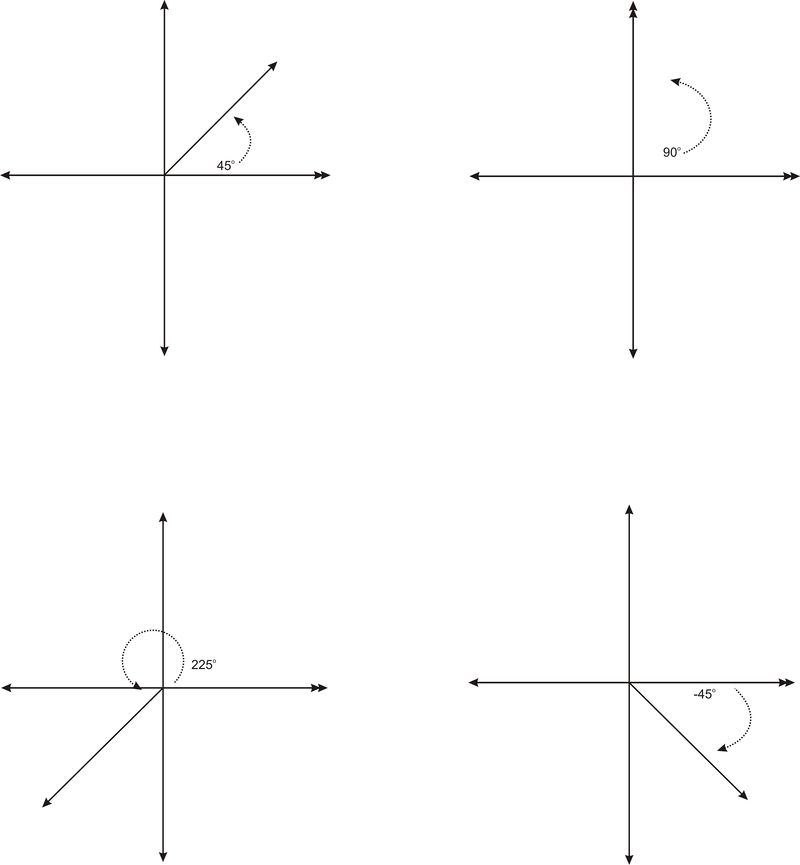The 90 degree angle is one of four quadrantal angles. A quadrantal angle is one whose terminal side lies on an axis. Along with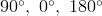and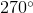are quadrantal angles.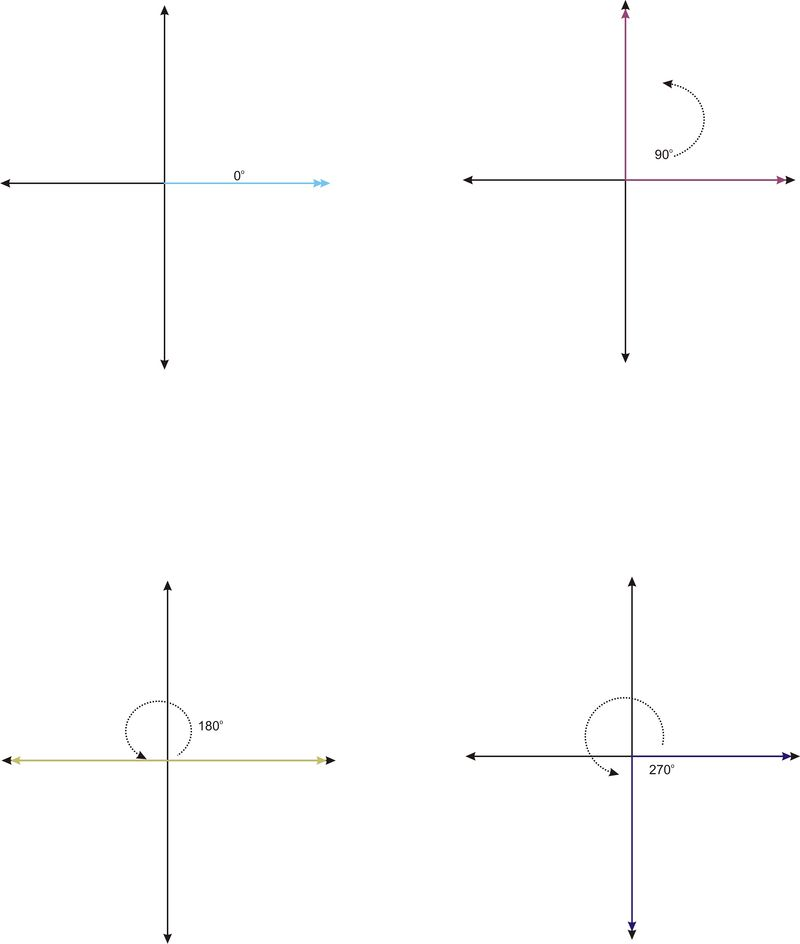These angles are referred to as quadrantal because each angle defines a quadrant. Notice that without the arrow indicating the rotation,looks as if it is a, defining the fourth quadrant. Notice also thatwould look just like.

#### Example A

Identify what the angle is in this graph: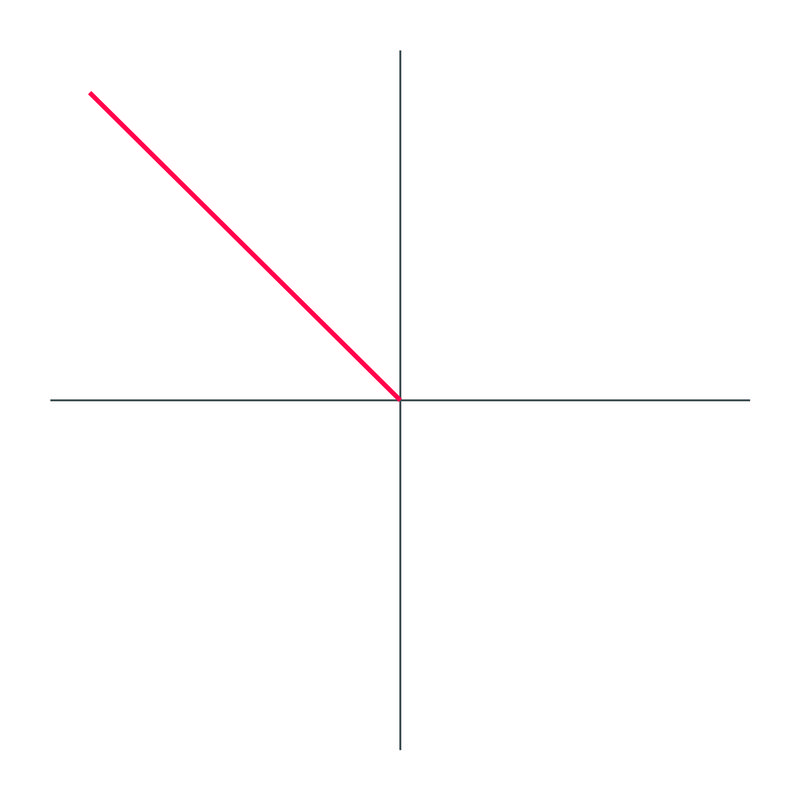Solution:

The angle drawn out is.

#### Example B

Identify what the angle is in this graph: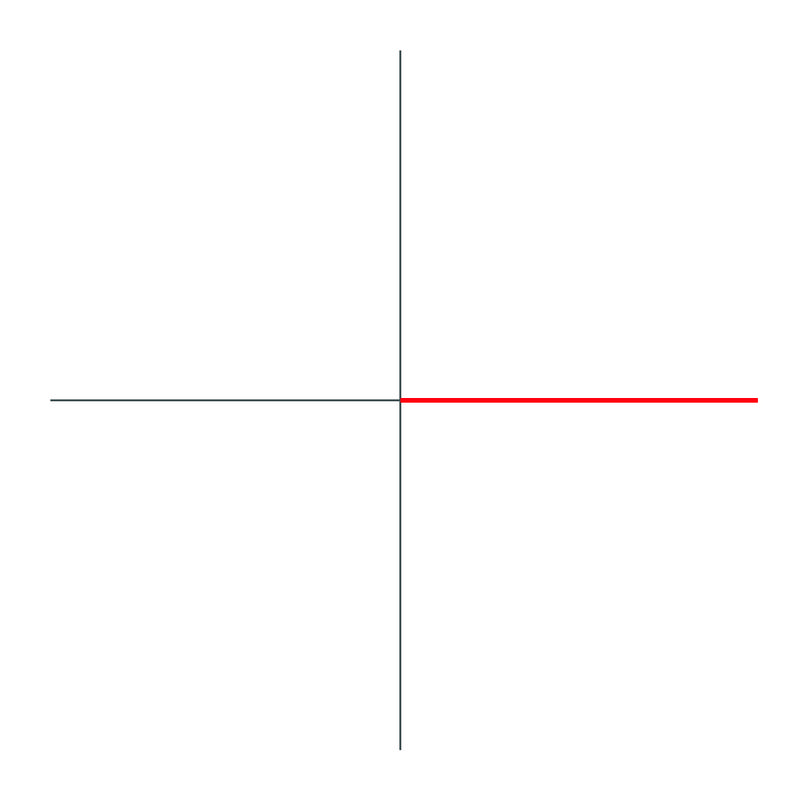Solution:

The angle drawn out is.

#### Example C

Identify what the angle is in this graph: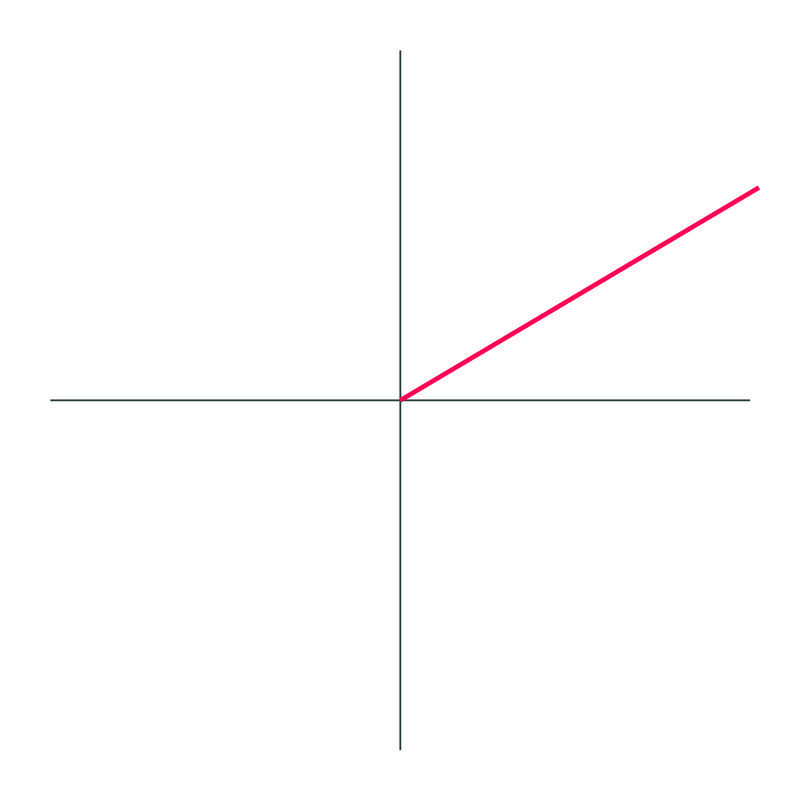Solution:

The angle drawn out is